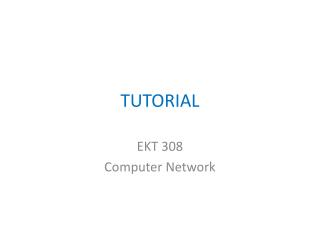Download PresentationTUTORIAL

# TUTORIAL - PowerPoint PPT Presentation

TUTORIAL. EKT 308 Computer Network. Question 1. 1. a) Differentiate between open loop and closed loop control systems. b) Explain positive features of feedback in a control system. c) Find poles and zeros of the overall transfer function of the systemI am the owner, or an agent authorized to act on behalf of the owner, of the copyrighted work described.
Download Presentation## TUTORIAL

Download Policy: Content on the Website is provided to you AS IS for your information and personal use and may not be sold / licensed / shared on other websites without getting consent from its author.While downloading, if for some reason you are not able to download a presentation, the publisher may have deleted the file from their server.

- - - - - - - - - - - - - - - - - - - - - - - - - - E N D - - - - - - - - - - - - - - - - - - - - - - - - - -
Presentation Transcript
1. TUTORIAL EKT 308 Computer Network

2. Question 1 1. a) Differentiate between open loop and closed loop control systems. b) Explain positive features of feedback in a control system. c) Find poles and zeros of the overall transfer function of the system in Figure 1. What is the output if a unit step input is applied?

3. 1. d) Figure 2.

4. Question 2 2. a) Find the state differential equation for the system in Figure 3. b) Find output. Figure 3.

5. 2. c) Find the stability of the following system 3. a) Define i) Rise time, ii) steady state error for a system for step input.

6. 3. b) If K = 20, find steady state error for the following system for ramp input. c) Steady state error for unit step. d) Find value of K for which percentage overshoot is 20% for unit step input.

7. SECTION B Question 1 A unity feedback system has the following open loop transfer function, Determine the range of K so that the closed loop system is stable. ii) Sketch the root locus for the above system.

8. Question 2 i) Consider the system in the following figure. Sketch the root locus for the above system. Find minimum jeta for stable complex root. Explain transfer function of a PID controller.

9. Question 3

10. Question 4

11. Question 5 ii) Draw an RC circuit that would function as a lead-lag network.